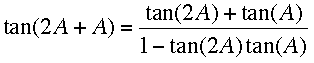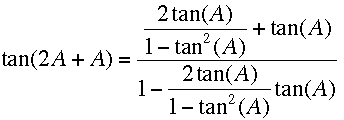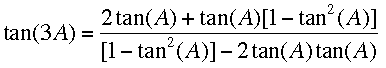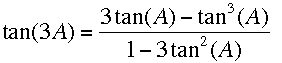SEARCH HOMEMath Central Quandaries & QueriesQuestion from sam, a student: Hi I am trying to derive a triple angle formulae for tan. I know i need to use compound and double angle formulae but am finding it difficult to "clean" up my fraction to get the triple angle formulae can you show me a worked derivation?! thanksHi Sam,

I am going to assume that you know the expressions for sine, cosine and tangent of A + B. There is some information in our answer to an earlier question.

Start by writing tan(3A) as tan(2A + A) and use the expression for tan(A + B) to getNow replace tan(2A) by 2tan(A)/(1-tan2(A))To simplify this expression, multiply the numerator and denominator by 1-tan2(A) and the expression becomesSimplification of this expression givesPennyMath Central is supported by the University of Regina and The Pacific Institute for the Mathematical Sciences.